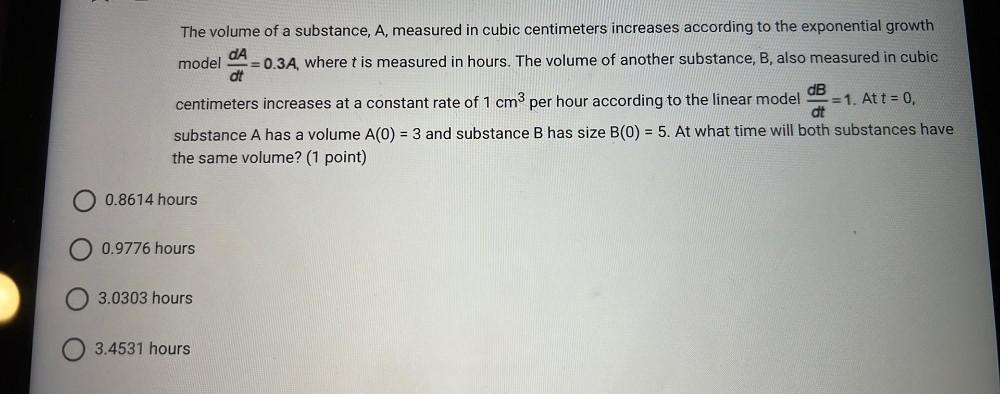Question:

# The volume of a substance, A, measured in cubic centimeters increases according to the exponential growth dA model =0.3A, whereThe volume of a substance, A, measured in cubic centimeters increases according to the exponential growth dA model =0.3A, where t is measured in hours. The volume of another substance, B, also measured in cubic dt dB centimeters increases at a constant rate of 1 cm3 per hour according to the linear model =1. At t = 0, dt substance A has a volume A(0) = 3 and substance B has size B(0) = 5. At what time will both substances have the same volume? (1 point) = = O 0.8614 hours O 0.9776 hours 3.0303 hours 0 3.4531 hours